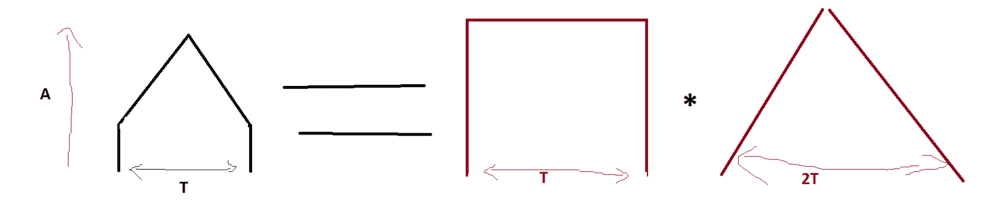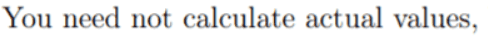# Fourier transform of pulses

• Electrical Engi321
Electrical Engi321
Hi,

In class I have learned how to find the Fourier transform of rectangular pulses. However, how do I solve a problem when I should sketch the Fourier transform of a pulse that isn't exactly rectangular.

For instance "Sketch the Fourier transform of the following 2 pulses"

Thanks in advance, I would have known how to exactly solve the problem if it was rectangular :(

#### Attachments

•fourierbild.PNG
23.8 KB · Views: 102

Homework Helper
Hello EE,!

In class I have learned
You most likely have learned a few things more - they are required for this exercise:
1. FT is linear: FT of a sum is sum of FT's and vice versa
2. FT of a product is a convolution and vice versa
And the exercise consists of recognizing which is which.

Oh, and this kind of exercise qualifies as homework in PF. For which we have

so next time we'll need a genuine effort (attempt at solution) from you•anorlunda
Electrical Engi321
Do you mean that g1 is the convolution of a linear function overlapped with a rectangle pusle? On both the negative and positive axis?

Homework Helper
Almost, but: it is not. Did you follow my simple advice ? What do you get when you convolve two rectangles ?

Electrical Engi321
One way to do this could be to look at the derivative two times, and graph the dirac amplitudes, then you get dirac functions as amplitudes (for the second derivative) then you conduct the Fourier of a derivative twice (iw)^2 (on the left hand side) and on the right hand side then you get dirac functions which become e^... Then you divide both sides with (iw)^2 and get the X(w) = ... e^... divided by (iw)^2 which you can graph in your calculator and hence make a sketch.

However that is when you do a Fourier with the variable w right (omega) now we have the Fourier transform in f where f=2*pi*w

Do you understand my concern, or do the way I suggested also work to draw the Fourier (instead of thinking in convolutions)?

Electrical Engi321
Almost, but: it is not. Did you follow my simple advice ? What do you get when you convolve two rectangles ?
You probably mean that you get a triangle when you convoluted two rectangles? I still don't really understand how this would help me. Can I use the sum of a 1. Rectanle pulse Fourier transformed 2. Triangular pulse? So

F(Rectangle + Rectangle*Rectangle) sort of thing?

Homework Helper
You're getting there ! Carry on ...

Electrical Engi321
You're getting there ! Carry on ...
which of the 2 methods do you think is the best way to go forward? I made 2 posts in a row, you maybe missed the first.

Homework Helper
I reponded to #6. Never saw #5 -- looks very convoluted (pardon the pun).

•rude man
Electrical Engi321
okay, okay.

Is this correct?Electrical Engi321
Where the * representens convolution. So the left picture is the convolution of the 2 red graphs?

Homework Helper
No, unfortunately not correct. If a plus sign explains g1, then this convolution certainly does not.
Counter-indication: the jump in g1

Work out the right-hand side using the definition of a convolution to prove it for yourself. Not all that complicated and very enlightening.

---

Electrical Engi321
I am becoming a bit more lost now haha.

The problem I think that I have hard to understand is that we are suppose to find the Fourier transform in Frequency domain and not omega. I were to set up an easy equation using heaviside and dirac to express this graph and use wolfram to find the Fourier it wouldn't give me it in Frequency domain. This is what makes me stuck I reckon

Homework Helper
You have seen that a convolution of two rectangles gives a triangle. Spend a few moment trying to understand why the convolution of a triangle and a rectangle should involve some ##t^2## -- so this does not appear in your exercise.

If that is asking too much, you can have it chewed out for you here (but I really think you should try it for yourself first
F(Rectangle + Rectangle*Rectangle) sort of thing?
Not 'sort of', but spot on !

----

Bedtime for me so I'll leave you to do g2 on your own. Ask yourself: if I get a triangle from two identical squares, what has to change to get a flat ceiling ?

Oh, and Wolfram is a nice crutch, but you want to learn to walk and run without

Staff Emeritus
I am becoming a bit more lost now haha.

The problem I think that I have hard to understand is that we are suppose to find the Fourier transform in Frequency domain and not omega. I were to set up an easy equation using heaviside and dirac to express this graph and use wolfram to find the Fourier it wouldn't give me it in Frequency domain. This is what makes me stuck I reckon

I'm confused on what the issue here is, and really confounded on why this has gone into the issue of convolution.

Presuming that you know the general equation of transforming from spatial to frequency domain, what exactly is the problem here?

The functions you were given in the attachments are symmetric, so you only need to multiply the function that you made with a cos function and integrate from 0 to ∞.

And oh, you definitely should know that ω = 2πf.

Is the source of the problem here is that you are unable to come up with the appropriate function in the first place?

Zz.

Homework Helper
I'm confused on what the issue here is, and really confounded on why this has gone into the issue of convolution.

Presuming that you know the general equation of transforming from spatial to frequency domain, what exactly is the problem here?

The functions you were given in the attachments are symmetric, so you only need to multiply the function that you made with a cos function and integrate from 0 to ∞.

And oh, you definitely should know that ω = 2πf.

Hello Zz,

I have the distinct impression that the purpose of this exercise is that the student should learn how to make good use of the linearity and the convolution theorem. True, I don't have the textbook and I can't read the writer's mind, but still:

Summary:: Finding Fourier transform of pulses that are not rectangular.

In class I have learned how to find the Fourier transform of rectangular pulses
...
Sketch the Fourier transform
...And of course the fact that the given shapes are so easily obtained from rectangular

The reactions of EE have not given me reason to change opinion. It is clear that he/she is still in an early stage of the curriculum for this most relevant topic.